4.10 The Answer Editor for Graphing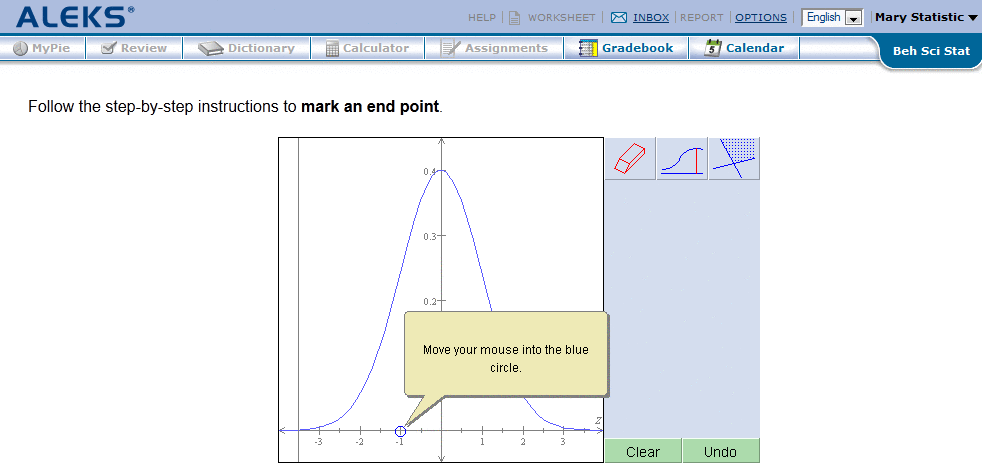Figure 4.4: The Answer Editor for Graphing
The Answer Editor for graphing consists of a Cartesian plane with x- and y- coordinate axes and a selection of other tools for graphing lines and regions of the plane (Fig. 4.4).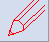To graph a line, use the pencil tool to plot two points. Then, align the straightedge (ruler) on the two points (it is a "grabby" tool and will jump to a point when it is near it). Then use the pencil tool to draw the line. Note that the effect of the straightedge continues past its ends, so there is no need to move it to make a line going from edge to edge of the depicted plane. The line should be started within the graph area, however.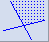To fill in a region, first, draw all the lines defining the region. Then use the region tool and click in the desired region of the plane. In order for one or more of the lines defining a region to be dotted (as in the graph of a system containing one or more strict inequalities), click on the line with the dotted line tool. This may be done before or after the region is filled.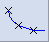To draw a graph, use the pencil tool to plot a point. Then, click on the Plot point button twice.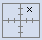To plot a point where the coordinates are non-integers, use the Plot point button. Using the keyboard, type the numerical values into the coordinate boxes and click Plot point.To draw a graph requiring an asymptote, use the asymptote tool (broken horizontal or vertical line) to place the asymptote as needed. A slanted asymptote may be placed by first drawing two points and then using the tool with a broken diagonal line. Plot the additional points needed for the graph, and then click on the graph button (curved line connecting "X"s).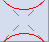For each type of conic section, there is a special tool allowing the construction of its graph. Normally, the user clicks once with the tool to establish the center or vertex of the graph, and then one or more additional times to determine its final form.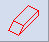As with the numberline, select the eraser tool and click on any part of a line, arc, or other component to remove it.